Like   Tweet   in# President's Note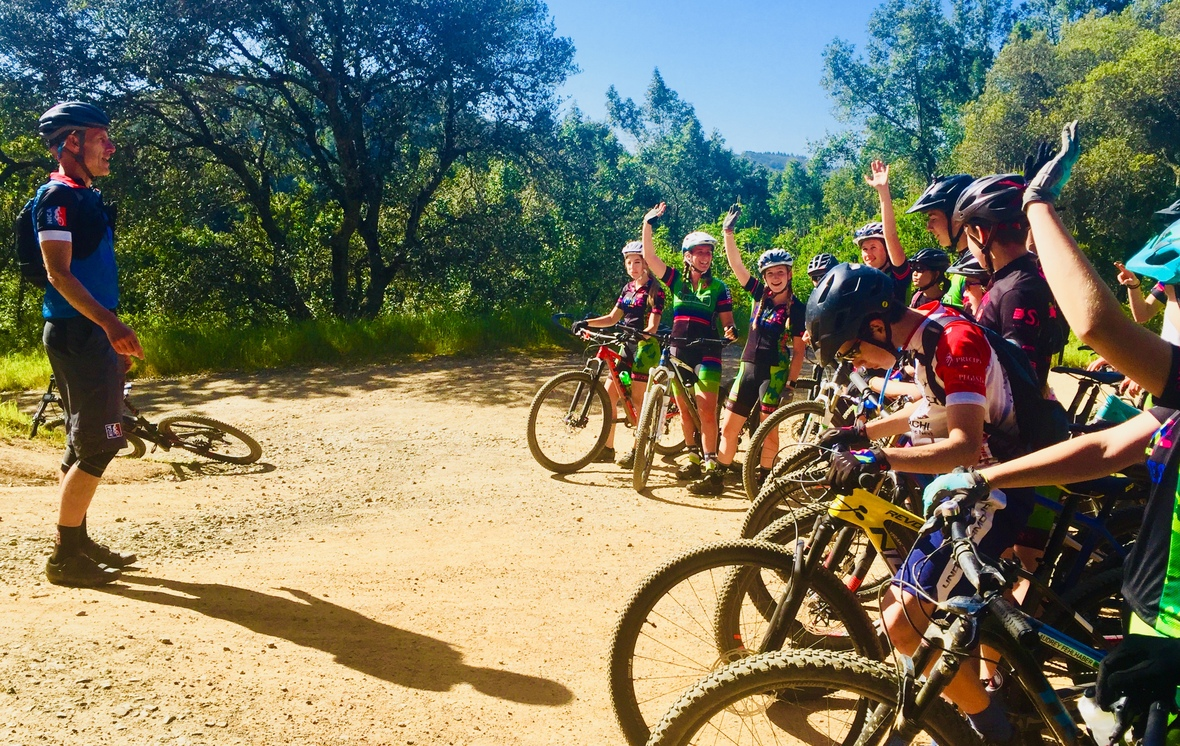Getting the Drake Pirates stoked before a team practice in NorCal!

As NICA continues to grow from coast to coast, it’s exciting to see how mountain biking brings student-athletes together not just as racers, but as a growing community of young adults that fully embody NICA’s 5 Core Principles of Equality, Inclusivity, Strong Mind, Strong Body and Strong Character.

This is made abundantly clear from the winners of our just completed Dirt Rag Magazine essay contest that asked student-athletes to detail how their coaches have helped them develop strong character. Picking finalists was an incredibly difficult decision as every essay submitted detailed the many different ways that interscholastic cycling is helping youth develop positive life skills both on and off the bike. You can meet the winners and read their essays further on in this edition of NICA News.

Earlier this month I also attended the Sea Otter Classic in Monterey and was blown away by the number of student-athletes racing and spectating. The size of the youth fields has been steadily increasing since NICA launched and it’s always exciting to catch-up with student-athletes and their families at the Sea Otter Expo! Equally as impressive was the size of the Pro Women’s cross country mountain bike race field where a significant portion of the athletes had connections with NICA either as alumnae or as current coaches.

During my recent travels, I have had the fortune to ride with five different high school teams and have been impressed to see that a number of NICA league grads who have completed college have returned to their hometowns to coach their old teams. NICA is not just getting #morekidsonbikes, we're getting more people on bikes across the county. As the stories and images in this month's NICA News will show - our programs continue to grow and diversify!

Sincerely,
Austin

 table div table+table+table+table div table{width:100%;padding:0}table div table+table+table+table div table img{width:96.23%;padding:0;float:none}table div table+table+table+table div table td{width:100%;padding:0 1.88% 18px}/* styles */# What's Happening Across America

## Spring League Racing

With New Jersey and New York kicking off their seasons this month, there are over 4,500 student-athletes racing across NorCal, SoCal,Texas, Alabama, North Carolina, New Jersey and New York. Congratulations to the Texas League on their State Championships April 21st and stay tuned for updates from other spring league state championships next month!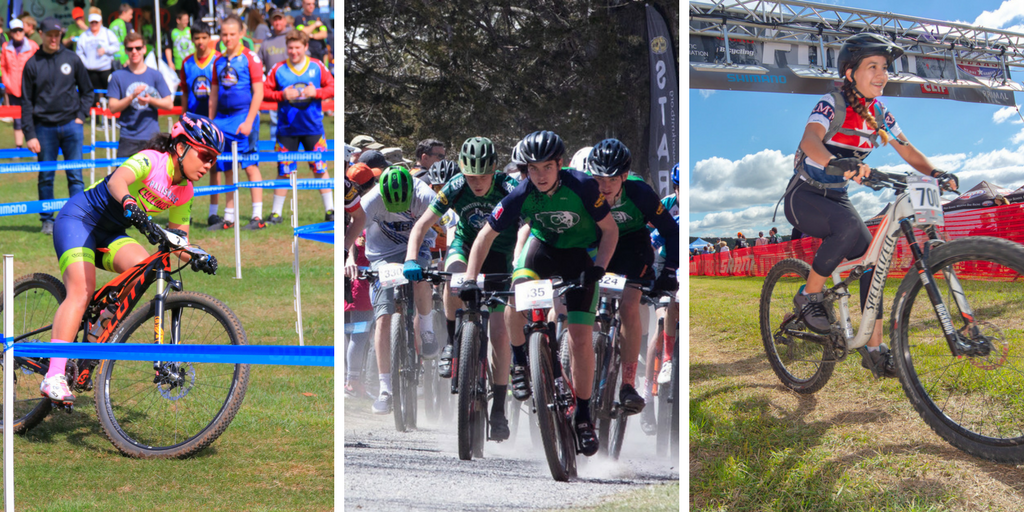New Jersey, New York and Texas Racing action. Photos: Don Madsen, Neil Goldberg and Steve Morton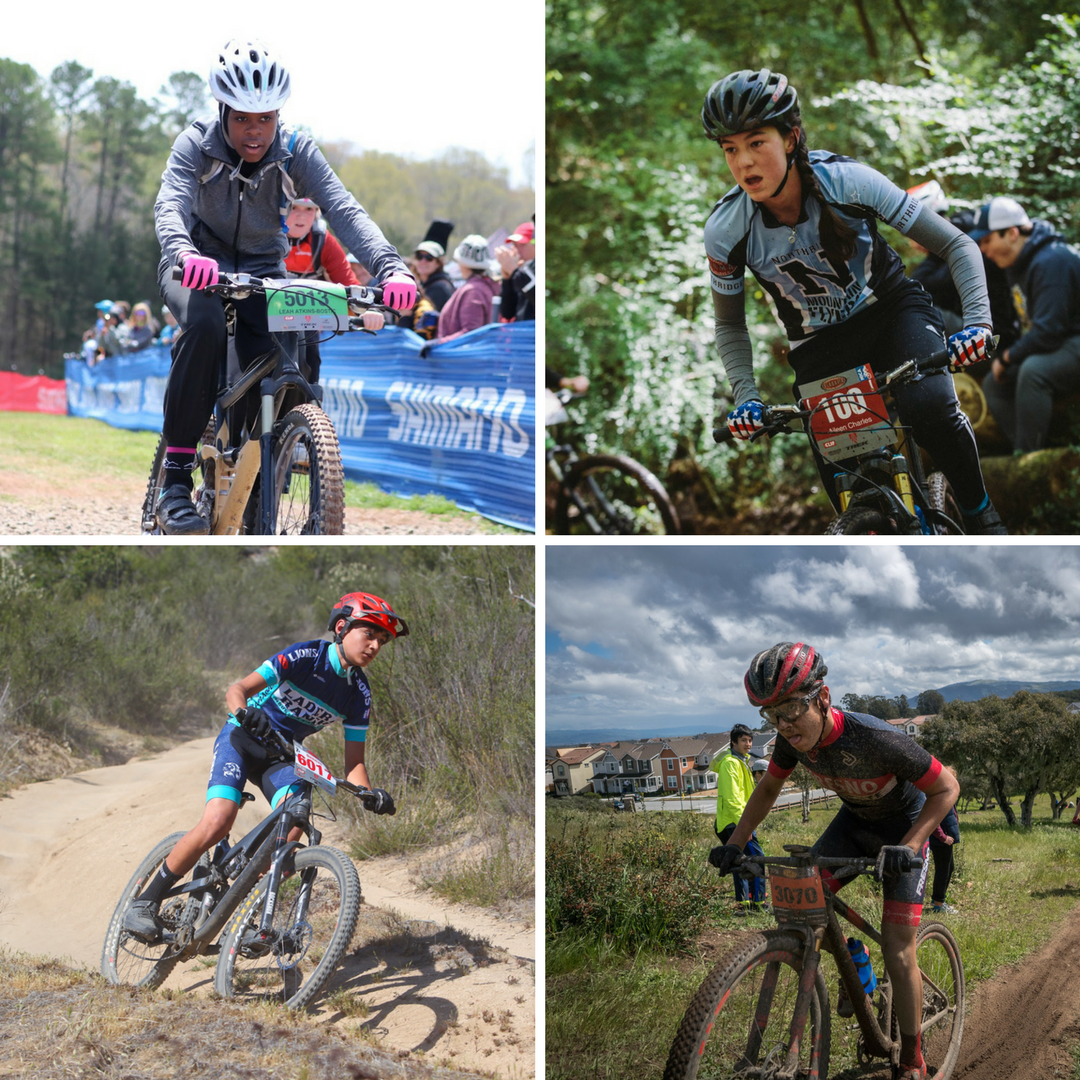North Carolina, Alabama, SoCal and NorCal Racing. Photos: Deborah Hague, Alabama NICA Race Media, Motovation Sports, Tim Madding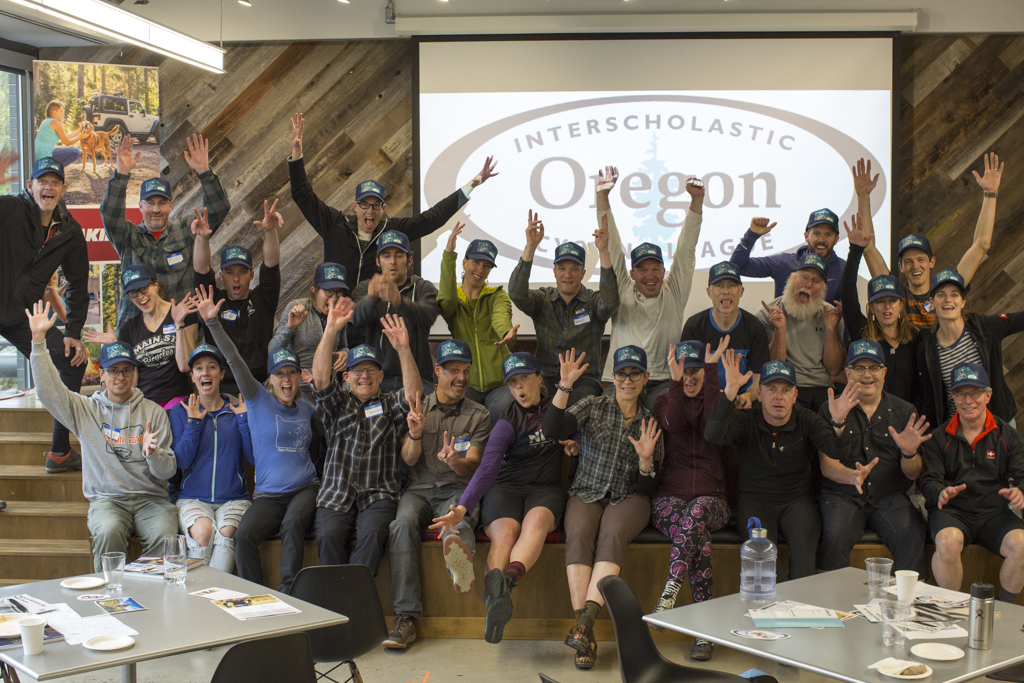The Inaugural Oregon League Leaders' Summit. Photo: Daniel Sharp

## Oregon Leaders' Summit

The Oregon League's inaugural Leaders' Summit took place at Yakima headquarters in Portland, Oregon, April 14-15 with over 30 coaches participating! Thank you to Yakima for hosting this successful and inspiring event, and a huge congratulation to Leauge Director Heather Wolfgang and new NICA Oregon Coaches for making this happen. Bravo! Oregon Coaches are equipped to bring interscholastic cycling to their state.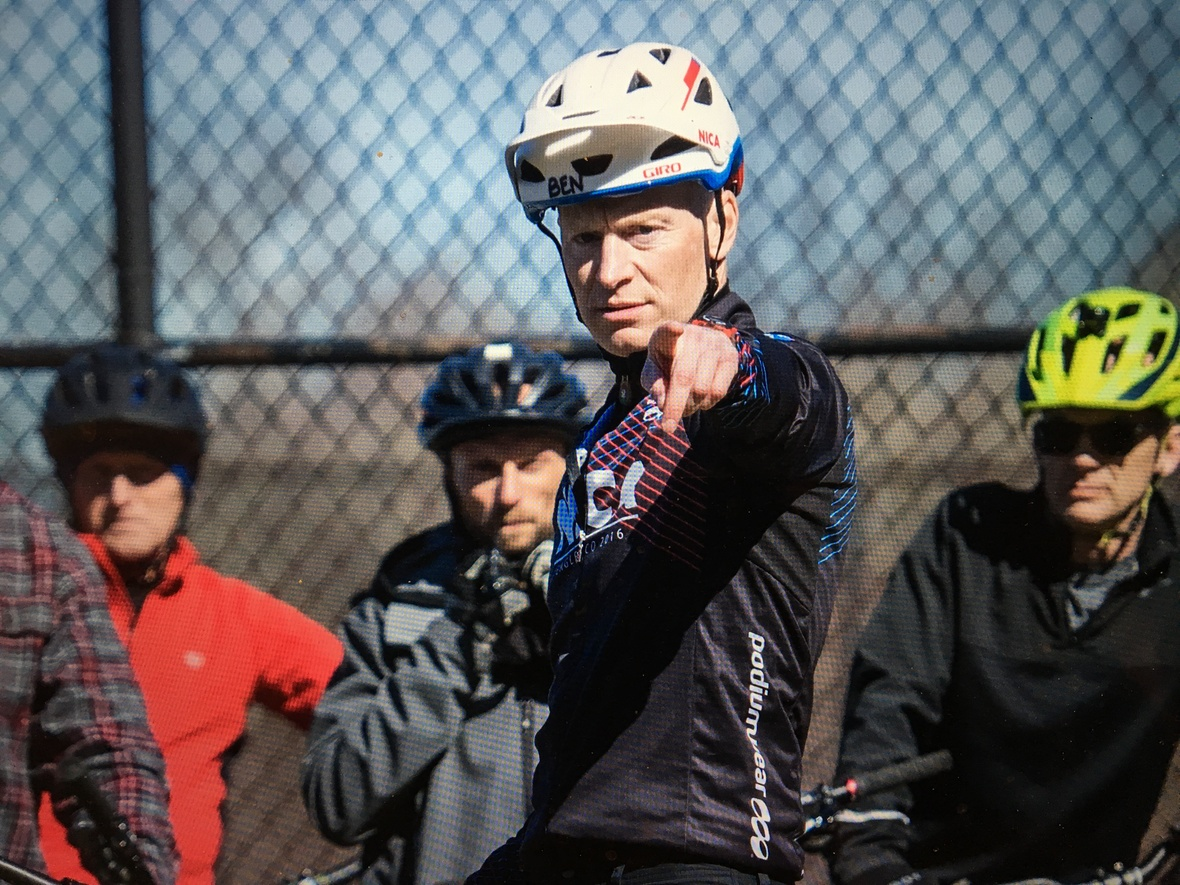NICA Coach Supporter Ben Tufford wants YOU to do the Spring 2018 Survey!

## We need YOU to complete our Spring League Survey!

Attention Spring Leagues! We need you (coaches, parents, student-athletes!) to complete our annual survey. You can access the survey HERE. This only takes a few moments of your time, but provides us with valuable information that we use to improve our programming. And don't worry - we will never share your personal data!

 table div table+table+table+table+table+table+table+table+table+table+table div table{width:100%;padding:0}table div table+table+table+table+table+table+table+table+table+table+table div table img{width:96.23%;padding:0;float:none}table div table+table+table+table+table+table+table+table+table+table+table div table td{width:100%;padding:0 1.88% 18px}/* styles */# NICA - Dirt Rag Student-Athlete Essay Contest

## Congratulations to our Winners!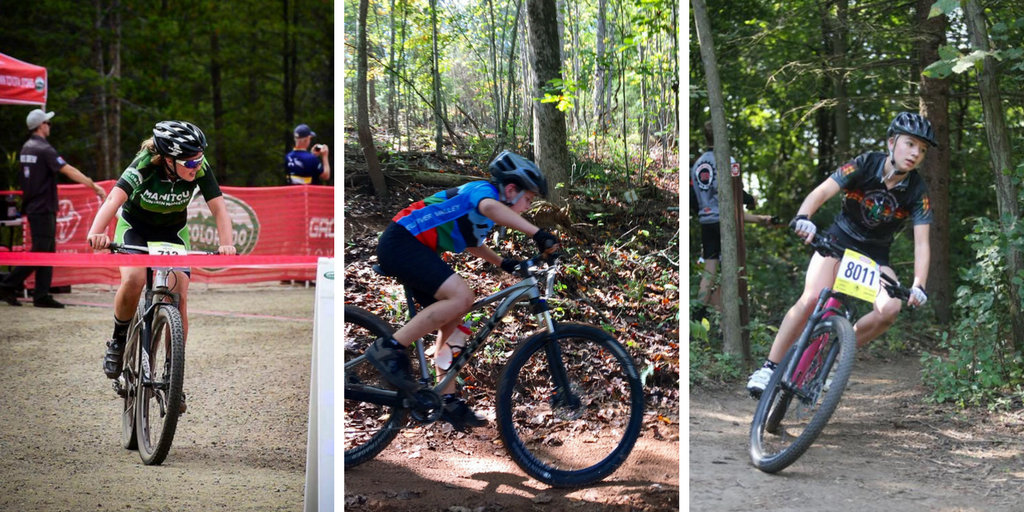Heidi Martin, Griffin Clubb and Sage Flory.
 /* styles */ Last month we announced an Essay Contest with Dirt Rag magazine where we asked student-athletes to tell us how they have been able to develop strong character through interscholastic cycling with the support of their coach. We received MANY wonderful entries and after much deliberation selected three winners. Congratulations to Heidi Martin from the Colorado League, Griffin Clubb from the Virginia League and Sage Flory from the Wisconsin League for their outstanding essays! Read their winning essays HERE.
 table div table+table+table+table+table+table+table+table+table+table+table+table+table+table+table div table{width:100%;padding:0}table div table+table+table+table+table+table+table+table+table+table+table+table+table+table+table div table img{width:96.23%;padding:0;float:none}table div table+table+table+table+table+table+table+table+table+table+table+table+table+table+table div table td{width:100%;padding:0 1.88% 18px}/* styles */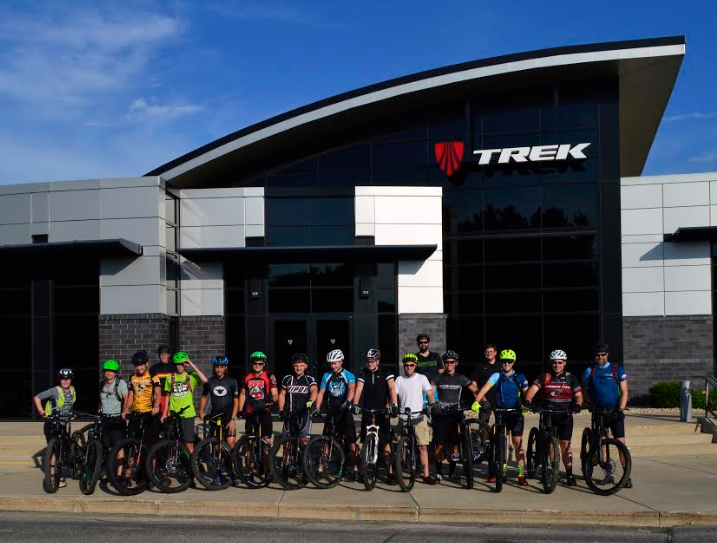Course participants get ready to ride the trails at Trek's headquarters in Waterloo, WI.

# Trek Certified Service Course

Trek Bicycle is once again offering their Assembly Technician 101 Course specifically for NICA student-athletes and Coaches this summer! They have three courses available in June and July. This is a great opportunity to learn bike assembly and mechanical skills AND a chance to ride the trails at Trek's Waterloo, WI headquarters! Details available HERE. Register soon as courses are filling quickly!

 table div table+table+table+table+table+table+table+table+table+table+table+table+table+table+table+table+table div table{width:100%;padding:0}table div table+table+table+table+table+table+table+table+table+table+table+table+table+table+table+table+table div table img{width:96.23%;padding:0;float:none}table div table+table+table+table+table+table+table+table+table+table+table+table+table+table+table+table+table div table td{width:100%;padding:0 1.88% 18px}/* styles */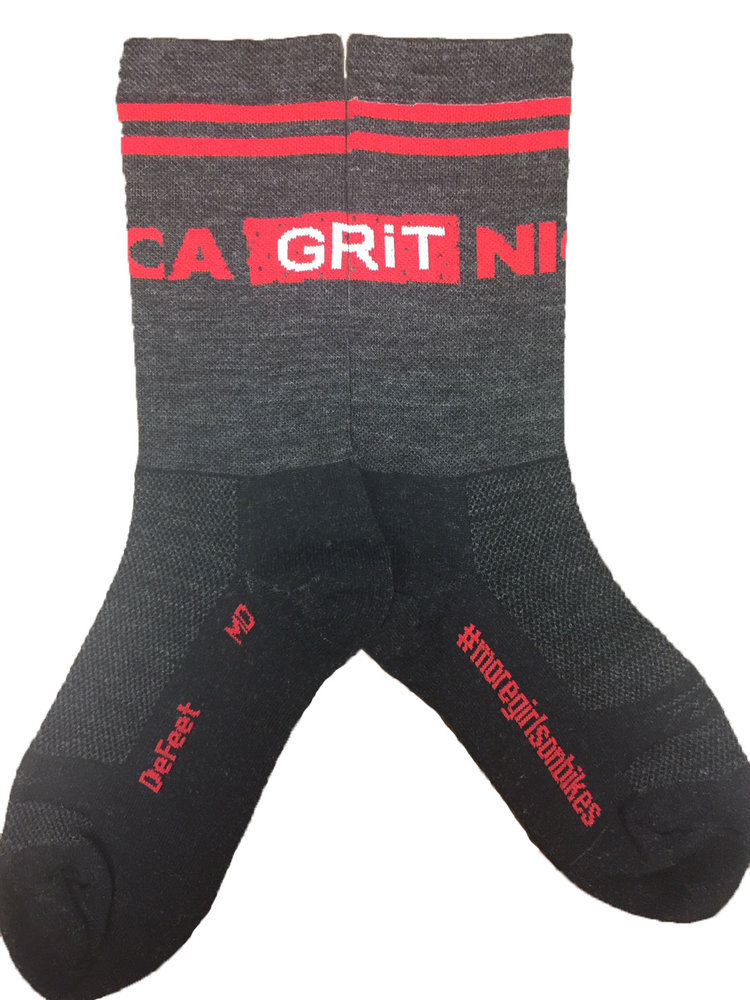# Get your NICA Gear On!

Have you visited the NICA Store lately? We've got some great new Podiumwear baggies available for men and women as well as free-ride jerseys and new NICA GRiT socks! Check it out and order yours today HERE.

 table div table+table+table+table+table+table+table+table+table+table+table+table+table+table+table+table+table+table+table div table{width:100%;padding:0}table div table+table+table+table+table+table+table+table+table+table+table+table+table+table+table+table+table+table+table div table img{width:96.23%;padding:0;float:none}table div table+table+table+table+table+table+table+table+table+table+table+table+table+table+table+table+table+table+table div table td{width:100%;padding:0 1.88% 18px}/* styles */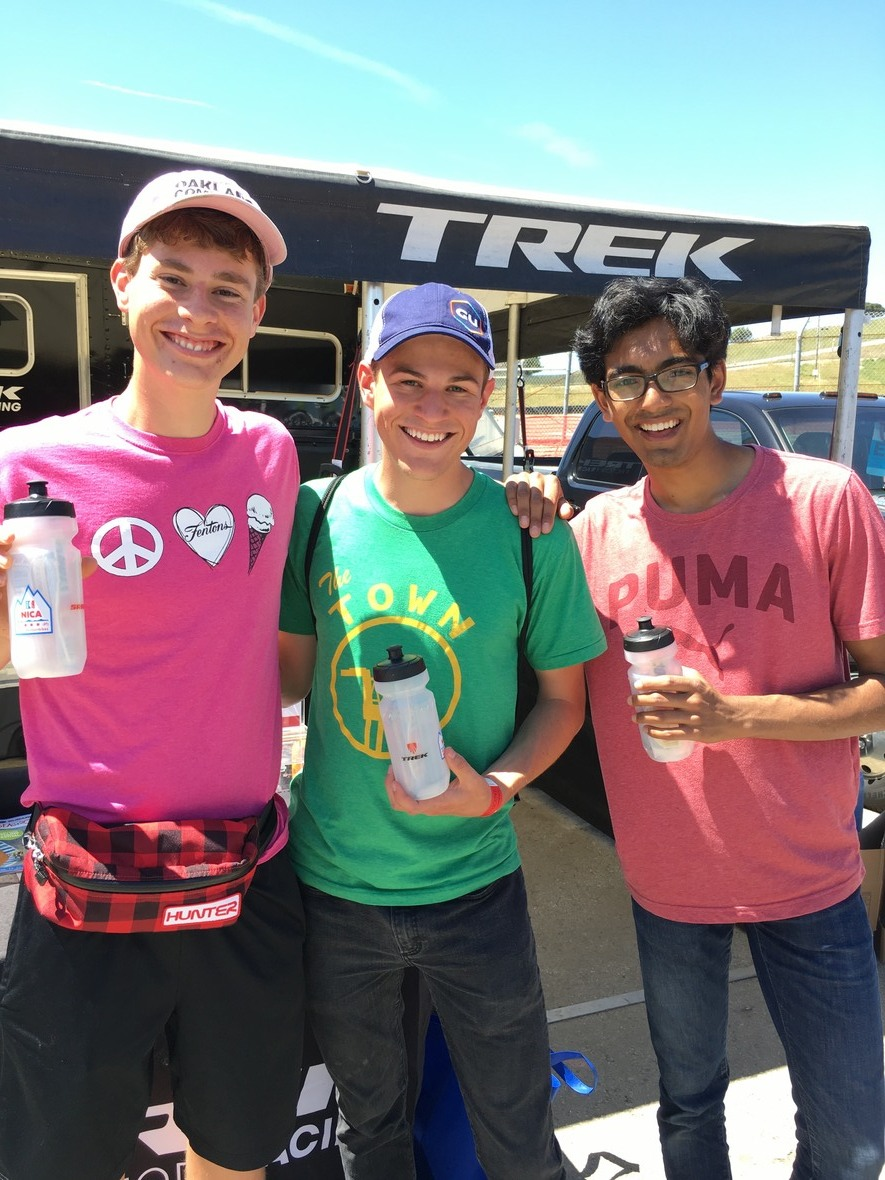# Thank You for a great Sea Otter Classic!

Thank you to all the student-athletes, their families and NICA sponsors and boosters who supported us and participated. in our scavenger hunt at this year's Sea Otter Classic! We hope everyone had a great time and are already looking forward to 2019!

Special thanks to the sponsors and boosters listed below who supported NICA through our scavenger hunt, social hours, race recovery lounges and sales! NICA raised nearly \$3,000 from custom Thule t-shirt sales and contributions made during a social hour jointly hosted by our friends at Park Tool & Bicycling Magazine!table div table+table+table+table+table+table+table+table+table+table+table+table+table+table+table+table+table+table+table+table+table+table div table{width:100%;padding:0}table div table+table+table+table+table+table+table+table+table+table+table+table+table+table+table+table+table+table+table+table+table+table div table img{width:96.23%;padding:0;float:none}table div table+table+table+table+table+table+table+table+table+table+table+table+table+table+table+table+table+table+table+table+table+table div table td{width:100%;padding:0 1.88% 18px}/* styles */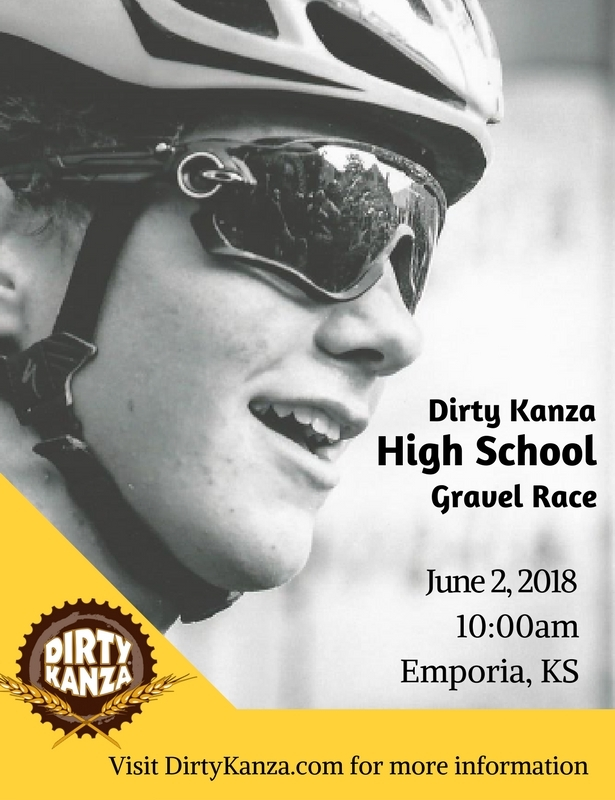# Dirty Kanza High School Race.

In an effort to bring interscholastic cycling to the midwest, Dirty Kanza is hosting a race specifically for high school students this year! NICA President, Austin McInerny and NorCal League Director, Vanessa Hauswald will be supporting the event, which includes a pre-race screening of our favorite, Singletrack High! More details and registration info on this fun event HERE. Join the fun!

 table div table+table+table+table+table+table+table+table+table+table+table+table+table+table+table+table+table+table+table+table+table+table+table+table div table{width:100%;padding:0}table div table+table+table+table+table+table+table+table+table+table+table+table+table+table+table+table+table+table+table+table+table+table+table+table div table img{width:96.23%;padding:0;float:none}table div table+table+table+table+table+table+table+table+table+table+table+table+table+table+table+table+table+table+table+table+table+table+table+table div table td{width:100%;padding:0 1.88% 18px}/* styles */# NICA in Your Neighborhood

## We LOVE seeing what our student-athletes, teams, coaches and leagues are up to across the country. Here are some of the highlights from the past month!

Do you have a great photo worthy of inclusion in a future issue of NICA News? If so, please email to media@nationalmtb.org and you may see it published! And don't forget - when you're posting your photos to tag @nationalmtb #morekidsonbikes for a chance to see your photo in NICA News!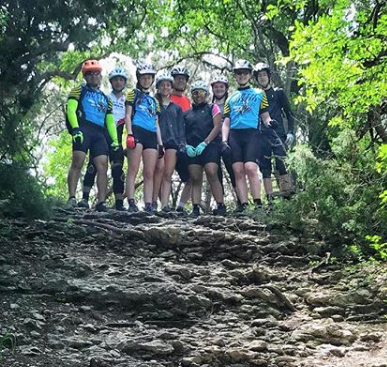Photo: Carlos Flores
 /* styles */ Coach @karlos_phlores and the North Austin T-Hawks pause for a mid-practice photo break while getting ready for the Texas State Championships!
 table.module-27{width:75.47%;padding:0}table div table+table+table+table+table+table+table+table+table+table+table+table+table+table+table+table+table+table+table+table+table+table+table+table+table+table+table+table div table{width:75.47%;float:none;margin-left:auto;margin-right:auto;padding:0}table div table+table+table+table+table+table+table+table+table+table+table+table+table+table+table+table+table+table+table+table+table+table+table+table+table+table+table+table div table a{border:0 none;text-decoration:none}table div table+table+table+table+table+table+table+table+table+table+table+table+table+table+table+table+table+table+table+table+table+table+table+table+table+table+table+table div table img{width:100%!important;border:0 none;text-decoration:none}table div table+table+table+table+table+table+table+table+table+table+table+table+table+table+table+table+table+table+table+table+table+table+table+table+table+table+table+table div table td{width:100%;padding:0}/* styles */
 /* styles */ The @sneaks_spokes Reactors braved the cold & wind at the Chester Challenge at Highlands Ridge Park for the first race of the 2018 @nicanewjersey season. Everyone did awesome for the 1st race of the season and we are looking forward to the next race on our home course at @campedgesj!
 table.module-29{width:75.47%;padding:0}table div table+table+table+table+table+table+table+table+table+table+table+table+table+table+table+table+table+table+table+table+table+table+table+table+table+table+table+table+table+table div table{width:75.47%;float:none;margin-left:auto;margin-right:auto;padding:0}table div table+table+table+table+table+table+table+table+table+table+table+table+table+table+table+table+table+table+table+table+table+table+table+table+table+table+table+table+table+table div table a{border:0 none;text-decoration:none}table div table+table+table+table+table+table+table+table+table+table+table+table+table+table+table+table+table+table+table+table+table+table+table+table+table+table+table+table+table+table div table img{width:100%!important;border:0 none;text-decoration:none}table div table+table+table+table+table+table+table+table+table+table+table+table+table+table+table+table+table+table+table+table+table+table+table+table+table+table+table+table+table+table div table td{width:100%;padding:0}/* styles */
 /* styles */ Yucaipa's @jrtbirdsmtb Coach Marino (aka Coach TeeMo) with first-year middle school rider, Missie Glen-Rosbrugh. It was a proud moment for all when Missie stepped onto the podium for 5th place at SoCal's Victory at Vail! #socalseasonx
 table.module-31{width:75.47%;padding:0}table div table+table+table+table+table+table+table+table+table+table+table+table+table+table+table+table+table+table+table+table+table+table+table+table+table+table+table+table+table+table+table+table div table{width:75.47%;float:none;margin-left:auto;margin-right:auto;padding:0}table div table+table+table+table+table+table+table+table+table+table+table+table+table+table+table+table+table+table+table+table+table+table+table+table+table+table+table+table+table+table+table+table div table a{border:0 none;text-decoration:none}table div table+table+table+table+table+table+table+table+table+table+table+table+table+table+table+table+table+table+table+table+table+table+table+table+table+table+table+table+table+table+table+table div table img{width:100%!important;border:0 none;text-decoration:none}table div table+table+table+table+table+table+table+table+table+table+table+table+table+table+table+table+table+table+table+table+table+table+table+table+table+table+table+table+table+table+table+table div table td{width:100%;padding:0}/* styles */
 /* styles */ @thehappyhuckeris stoked to see Nino Shorter in intense race mode on his way to a third place finish at the @northcarolinamtb race at Big Leaf Slopes!
 table.module-33{width:75.47%;padding:0}table div table+table+table+table+table+table+table+table+table+table+table+table+table+table+table+table+table+table+table+table+table+table+table+table+table+table+table+table+table+table+table+table+table+table div table{width:75.47%;float:none;margin-left:auto;margin-right:auto;padding:0}table div table+table+table+table+table+table+table+table+table+table+table+table+table+table+table+table+table+table+table+table+table+table+table+table+table+table+table+table+table+table+table+table+table+table div table a{border:0 none;text-decoration:none}table div table+table+table+table+table+table+table+table+table+table+table+table+table+table+table+table+table+table+table+table+table+table+table+table+table+table+table+table+table+table+table+table+table+table div table img{width:100%!important;border:0 none;text-decoration:none}table div table+table+table+table+table+table+table+table+table+table+table+table+table+table+table+table+table+table+table+table+table+table+table+table+table+table+table+table+table+table+table+table+table+table div table td{width:100%;padding:0}/* styles */
 /* styles */ Thanks to local @trekbikes rep Adam Lewandowski @marylandmtb co-Director Fred Powell was able to find his happy place. Taking a Full Stache out for a demo can change a man!
 table.module-35{width:75.47%;padding:0}table div table+table+table+table+table+table+table+table+table+table+table+table+table+table+table+table+table+table+table+table+table+table+table+table+table+table+table+table+table+table+table+table+table+table+table+table div table{width:75.47%;float:none;margin-left:auto;margin-right:auto;padding:0}table div table+table+table+table+table+table+table+table+table+table+table+table+table+table+table+table+table+table+table+table+table+table+table+table+table+table+table+table+table+table+table+table+table+table+table+table div table a{border:0 none;text-decoration:none}table div table+table+table+table+table+table+table+table+table+table+table+table+table+table+table+table+table+table+table+table+table+table+table+table+table+table+table+table+table+table+table+table+table+table+table+table div table img{width:100%!important;border:0 none;text-decoration:none}table div table+table+table+table+table+table+table+table+table+table+table+table+table+table+table+table+table+table+table+table+table+table+table+table+table+table+table+table+table+table+table+table+table+table+table+table div table td{width:100%;padding:0}/* styles */
 /* styles */ @zuintheozarks got a lot of insights into coaching a NICA team at the @arkansasmtb Leaders' Summit earlier this month!
 table div table+table+table+table+table+table+table+table+table+table+table+table+table+table+table+table+table+table+table+table+table+table+table+table+table+table+table+table+table+table+table+table+table+table+table+table+table+table div table{width:100%;padding:0}table div table+table+table+table+table+table+table+table+table+table+table+table+table+table+table+table+table+table+table+table+table+table+table+table+table+table+table+table+table+table+table+table+table+table+table+table+table+table div table img{width:96.23%;padding:0;float:none}table div table+table+table+table+table+table+table+table+table+table+table+table+table+table+table+table+table+table+table+table+table+table+table+table+table+table+table+table+table+table+table+table+table+table+table+table+table+table div table td{width:100%;padding:0 1.88% 18px}/* styles */